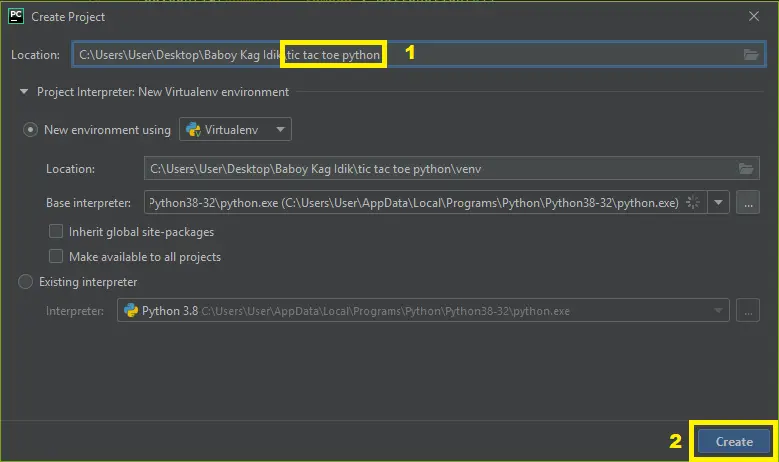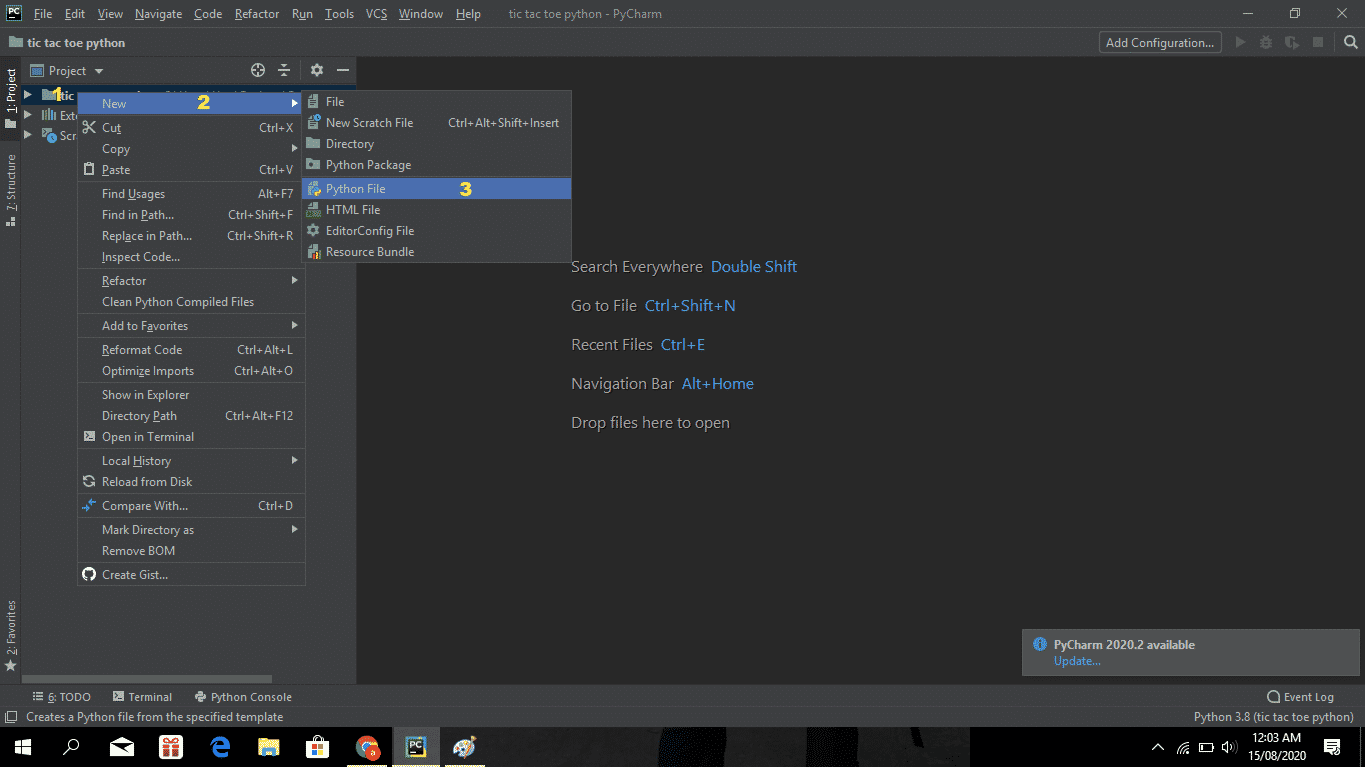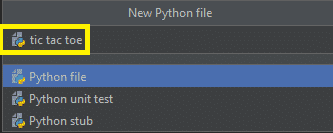# Tic Tac Toe Game In Python With Source Code

## Tic Tac Toe Game In Python With Source Code

The Tic Tac Toe Game In Python is written in python programming language, This Tic Tac Toe In Python is a simple GUI based strategy board game that is very easy to understand and use. All the playing rules are the same just like we play in real time tic-tac-toe. This Tic Tac Toe Python Program is a simple multiplayer game.

A Tic Tac Toe In Python Using Tkinter game starts with a GUI board. First, the player has to click on any box to start the game. It contains the Minimax algorithm; which is a decision rule used for a two-player game. A simple Tic Tac Toe Python Code 2 Player 2D GUI is provided for easy gameplay. The gameplay design is so simple that user won’t find it difficult to use and understand.

Anyway if you want level up your knowledge in programming especially games in python, try this new article I’ve made for you Code For Game in Python: Python Game Projects With Source Code

Tic Tac Toe Game in Python with Sou...
Tic Tac Toe Game in Python with Source Code 2020 Free Download

To start creating a Tic Tac Toe Game In Python, make sure that you have PyCharm IDE installed in your computer.

By the way if you are new to python programming and you don’t know what would be the the Python IDE to use, I have here a list of Best Python IDE for Windows, Linux, Mac OS that will suit for you. I also have here How to Download and Install Latest Version of Python on Windows.

## Steps on How To Create Tic Tac Toe In Python

Tic Tac Toe Game In Python With Source Code

• Step 1: Create a project name.

First open Pycharm IDE and then create a “project name” after creating a project name click the “create” button.• Step 2: Create a python file.

Second after creating a project name, “right click” your project name and then click “new” after that click the “python file“.• Step 3: Name your python file.

Third after creating a python file, Name your python file after that click “enter“.• Step 4: The actual code.

You are free to copy the code given below and download the full source code below.

## The Code Given Below Is For Importing Modules

``````from tkinter import Tk,ttk,Button
from tkinter import messagebox
from random import randint``````

The code given which is importing all modules or libraries.

## The Code Given Below Is For The Module Lay Out

``````def SetLayout(id,player_symbol):
if id==1:
b1.config(text= player_symbol)
b1.state(['disabled'])
elif id==2:
b2.config(text= player_symbol)
b2.state(['disabled'])
elif id==3:
b3.config(text= player_symbol)
b3.state(['disabled'])
elif id==4:
b4.config(text= player_symbol)
b4.state(['disabled'])
elif id==5:
b5.config(text= player_symbol)
b5.state(['disabled'])
elif id==6:
b6.config(text= player_symbol)
b6.state(['disabled'])
elif id==7:
b7.config(text= player_symbol)
b7.state(['disabled'])
elif id==8:
b8.config(text= player_symbol)
b8.state(['disabled'])
elif id==9:
b9.config(text= player_symbol)
b9.state(['disabled'])``````

In this module which is setting the layout of its player symbol.

## The Code Given Below Is For The Check Winner Module

``````def CheckWinner():
global mov
winner = -1

if(1 in p1) and (2 in p1) and (3 in p1):
winner = 1
if(1 in p2) and (2 in p2) and (3 in p2):
winner = 2

if(4 in p1) and (5 in p1) and (6 in p1):
winner = 1
if(4 in p2) and (5 in p2) and (6 in p2):
winner = 2

if(7 in p1) and (8 in p1) and (9 in p1):
winner = 1
if(7 in p2) and (8 in p2) and (9 in p2):
winner = 2

if(1 in p1) and (4 in p1) and (7 in p1):
winner = 1
if(1 in p2) and (4 in p2) and (7 in p2):
winner = 2

if(2 in p1) and (5 in p1) and (8 in p1):
winner = 1
if(2 in p2) and (5 in p2) and (8 in p2):
winner = 2

if(3 in p1) and (6 in p1) and ( 9 in p1):
winner = 1
if(3 in p2) and (6 in p2) and (9 in p2):
winner = 2

if(1 in p1) and (5 in p1) and ( 9 in p1):
winner = 1
if(1 in p2) and (5 in p2) and (9 in p2):
winner = 2

if(3 in p1) and (5 in p1) and ( 7 in p1):
winner = 1
if(3 in p2) and (5 in p2) and (7 in p2):
winner = 2

if winner ==1:
messagebox.showinfo(title="Congratulations.",
message="Player 1 is the winner")
elif winner ==2:
messagebox.showinfo(title="Congratulations.",
message="Player 2 is the winner")
elif mov ==9:
messagebox.showinfo(title="Draw",
message="It's a Draw!!")``````

In this module which is checking the winner of two players.

## The Code Given Below Is For The Module Button Click

``````def ButtonClick(id):
global ActivePlayer
global p1,p2
global mov

if(ActivePlayer ==1):
SetLayout(id,"X")
p1.append(id)
mov +=1
root.title("Tic Tac Toe : Player 2")
ActivePlayer =2

elif(ActivePlayer==2):
SetLayout(id,"O")
p2.append(id)
mov +=1
root.title("Tic Tac Toe : Player 1")
ActivePlayer =1
CheckWinner()``````

In this module which is button click of two players and its conditions.

## The Code Given Below Is For The Module Restart

``````def Restart():
global p1,p2,mov,ActivePlayer
p1.clear(); p2.clear()
mov,ActivePlayer = 0,1
root.title("Tic Tac Toe : Player 1")
EnableAll()``````

In this module which is the restarting or the new game module of the game.

## Complete Source Code

``````from tkinter import Tk,ttk,Button
from tkinter import messagebox
from random import randint

ActivePlayer = 1
p1 = []
p2 = []
mov = 0

def SetLayout(id,player_symbol):
if id==1:
b1.config(text= player_symbol)
b1.state(['disabled'])
elif id==2:
b2.config(text= player_symbol)
b2.state(['disabled'])
elif id==3:
b3.config(text= player_symbol)
b3.state(['disabled'])
elif id==4:
b4.config(text= player_symbol)
b4.state(['disabled'])
elif id==5:
b5.config(text= player_symbol)
b5.state(['disabled'])
elif id==6:
b6.config(text= player_symbol)
b6.state(['disabled'])
elif id==7:
b7.config(text= player_symbol)
b7.state(['disabled'])
elif id==8:
b8.config(text= player_symbol)
b8.state(['disabled'])
elif id==9:
b9.config(text= player_symbol)
b9.state(['disabled'])

def CheckWinner():
global mov
winner = -1

if(1 in p1) and (2 in p1) and (3 in p1):
winner = 1
if(1 in p2) and (2 in p2) and (3 in p2):
winner = 2

if(4 in p1) and (5 in p1) and (6 in p1):
winner = 1
if(4 in p2) and (5 in p2) and (6 in p2):
winner = 2

if(7 in p1) and (8 in p1) and (9 in p1):
winner = 1
if(7 in p2) and (8 in p2) and (9 in p2):
winner = 2

if(1 in p1) and (4 in p1) and (7 in p1):
winner = 1
if(1 in p2) and (4 in p2) and (7 in p2):
winner = 2

if(2 in p1) and (5 in p1) and (8 in p1):
winner = 1
if(2 in p2) and (5 in p2) and (8 in p2):
winner = 2

if(3 in p1) and (6 in p1) and ( 9 in p1):
winner = 1
if(3 in p2) and (6 in p2) and (9 in p2):
winner = 2

if(1 in p1) and (5 in p1) and ( 9 in p1):
winner = 1
if(1 in p2) and (5 in p2) and (9 in p2):
winner = 2

if(3 in p1) and (5 in p1) and ( 7 in p1):
winner = 1
if(3 in p2) and (5 in p2) and (7 in p2):
winner = 2

if winner ==1:
messagebox.showinfo(title="Congratulations.",
message="Player 1 is the winner")
elif winner ==2:
messagebox.showinfo(title="Congratulations.",
message="Player 2 is the winner")
elif mov ==9:
messagebox.showinfo(title="Draw",
message="It's a Draw!!")

def ButtonClick(id):
global ActivePlayer
global p1,p2
global mov

if(ActivePlayer ==1):
SetLayout(id,"X")
p1.append(id)
mov +=1
root.title("Tic Tac Toe : Player 2")
ActivePlayer =2

elif(ActivePlayer==2):
SetLayout(id,"O")
p2.append(id)
mov +=1
root.title("Tic Tac Toe : Player 1")
ActivePlayer =1
CheckWinner()

def AutoPlay():
global p1; global p2
Empty = []
for cell in range(9):
if(not((cell +1 in p1) or (cell +1 in p2))):
Empty.append(cell+1)
try:
RandIndex = randint(0,len(Empty) -1)
ButtonClick(Empty[RandIndex])
except:
pass

def EnableAll():
b1.config(text= " ")
b1.state(['!disabled'])
b2.config(text= " ")
b2.state(['!disabled'])
b3.config(text= " ")
b3.state(['!disabled'])
b4.config(text= " ")
b4.state(['!disabled'])
b5.config(text= " ")
b5.state(['!disabled'])
b6.config(text= " ")
b6.state(['!disabled'])
b7.config(text= " ")
b7.state(['!disabled'])
b8.config(text= " ")
b8.state(['!disabled'])
b9.config(text= " ")
b9.state(['!disabled'])

def Restart():
global p1,p2,mov,ActivePlayer
p1.clear(); p2.clear()
mov,ActivePlayer = 0,1
root.title("Tic Tac Toe : Player 1")
EnableAll()

root = Tk()
root.title("Tic Tac toe : Player 1")
st = ttk.Style()
st.configure("my.TButton", font=('Chiller',24,'bold'))

b1 = ttk.Button(root, text=" ", style="my.TButton")
b1.config(command = lambda : ButtonClick(1))

b2 = ttk.Button(root, text=" ",style ="my.TButton")
b2.config(command = lambda : ButtonClick(2))

b3= ttk.Button(root, text=" ",style="my.TButton")
b3.config(command = lambda : ButtonClick(3))

b4 = ttk.Button(root, text=" ",style="my.TButton")
b4.config(command = lambda : ButtonClick(4))

b5 = ttk.Button(root, text=" ",style="my.TButton")
b5.config(command = lambda : ButtonClick(5))

b6 = ttk.Button(root, text=" ",style="my.TButton")
b6.config(command = lambda : ButtonClick(6))

b7 = ttk.Button(root, text=" ",style="my.TButton")
b7.config(command = lambda : ButtonClick(7))

b8 = ttk.Button(root, text=" ",style="my.TButton")
b8.config(command = lambda : ButtonClick(8))

b9 = ttk.Button(root, text=" ",style="my.TButton")
b9.config(command = lambda : ButtonClick(9))

Button(text="New Game..", font=('Courier new', 22, 'bold'), bg='blue', fg='white',
border=5, width=4,command = lambda :Restart()).grid(row=0, column=1, sticky="we")
root.resizable(0,0)
root.mainloop()
``````

## Summary

The Tic Tac Toe Game In Python is written in Python programming language, Python is very smooth to research the syntax emphasizes readability and it is able to reduces time ingesting in developing.

This is a simple GUI Based system, specially written for the beginners. Tic Tac Toe in Python project with source code is free to download. Use for education purpose only!

Also in this tutorial is the simplest way for the beginners or the student to enhance their logical skills in programming. and also in this game project is the way for the students or beginners in designing and developing games.